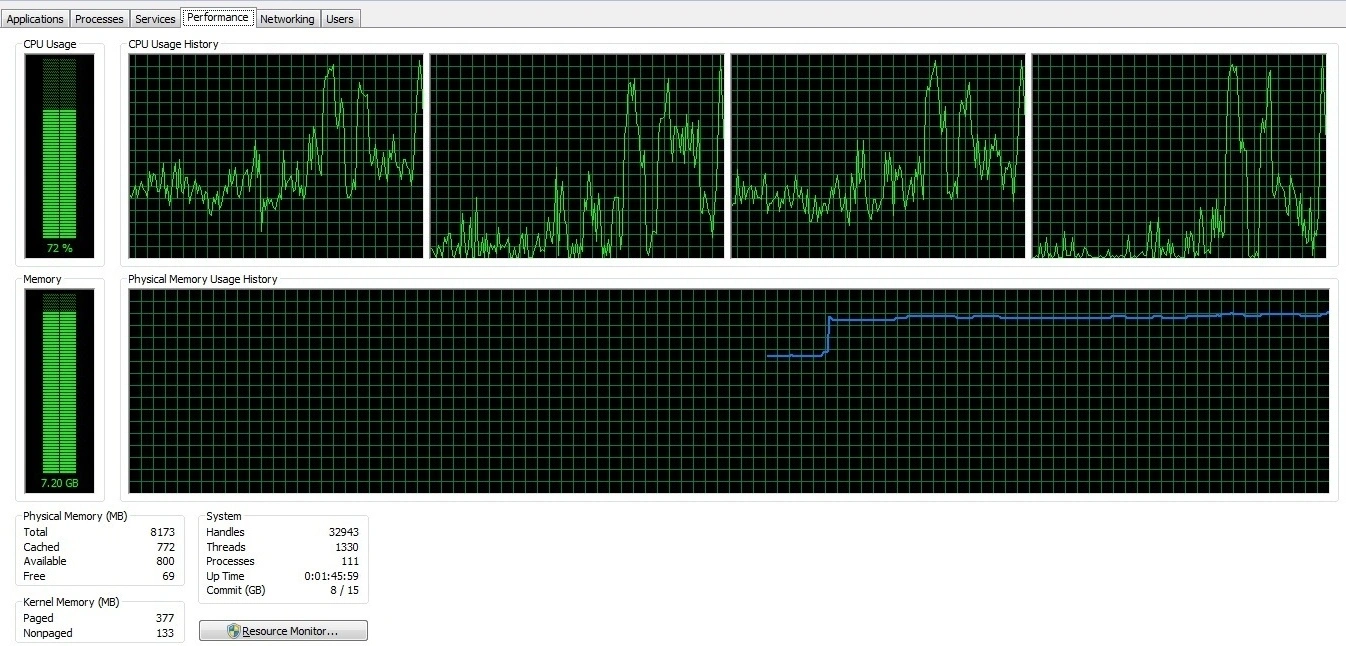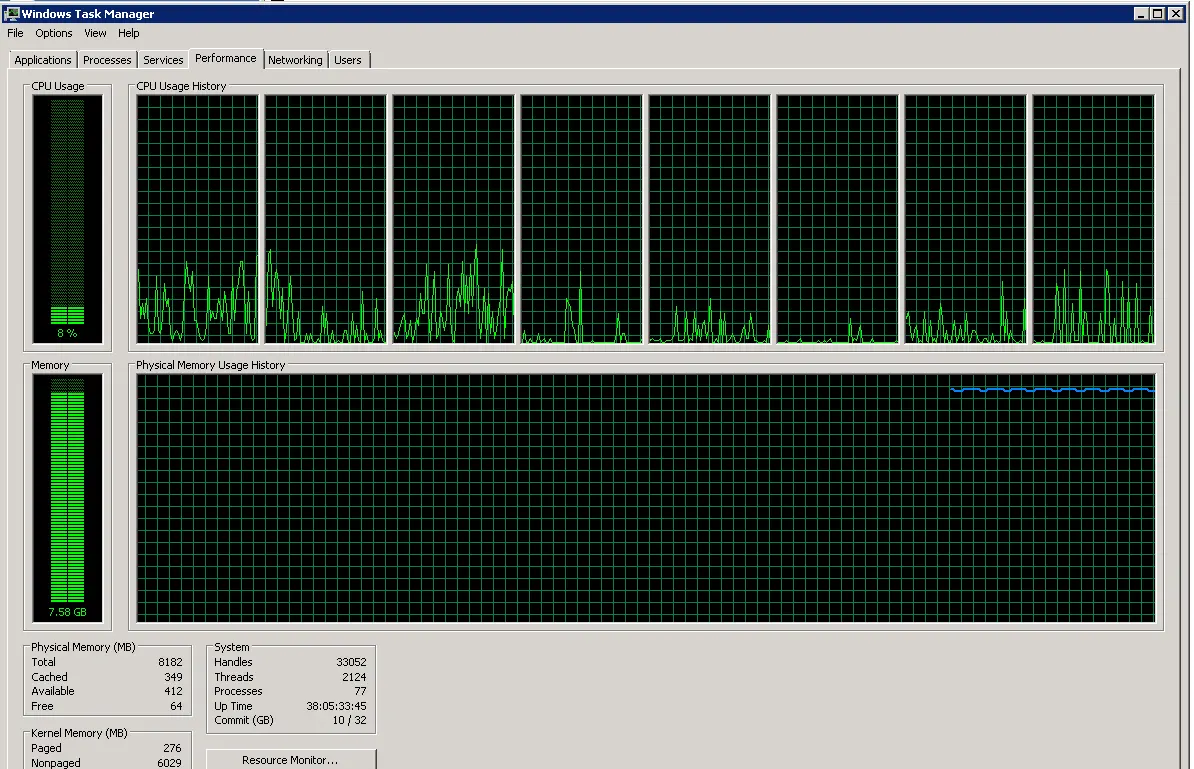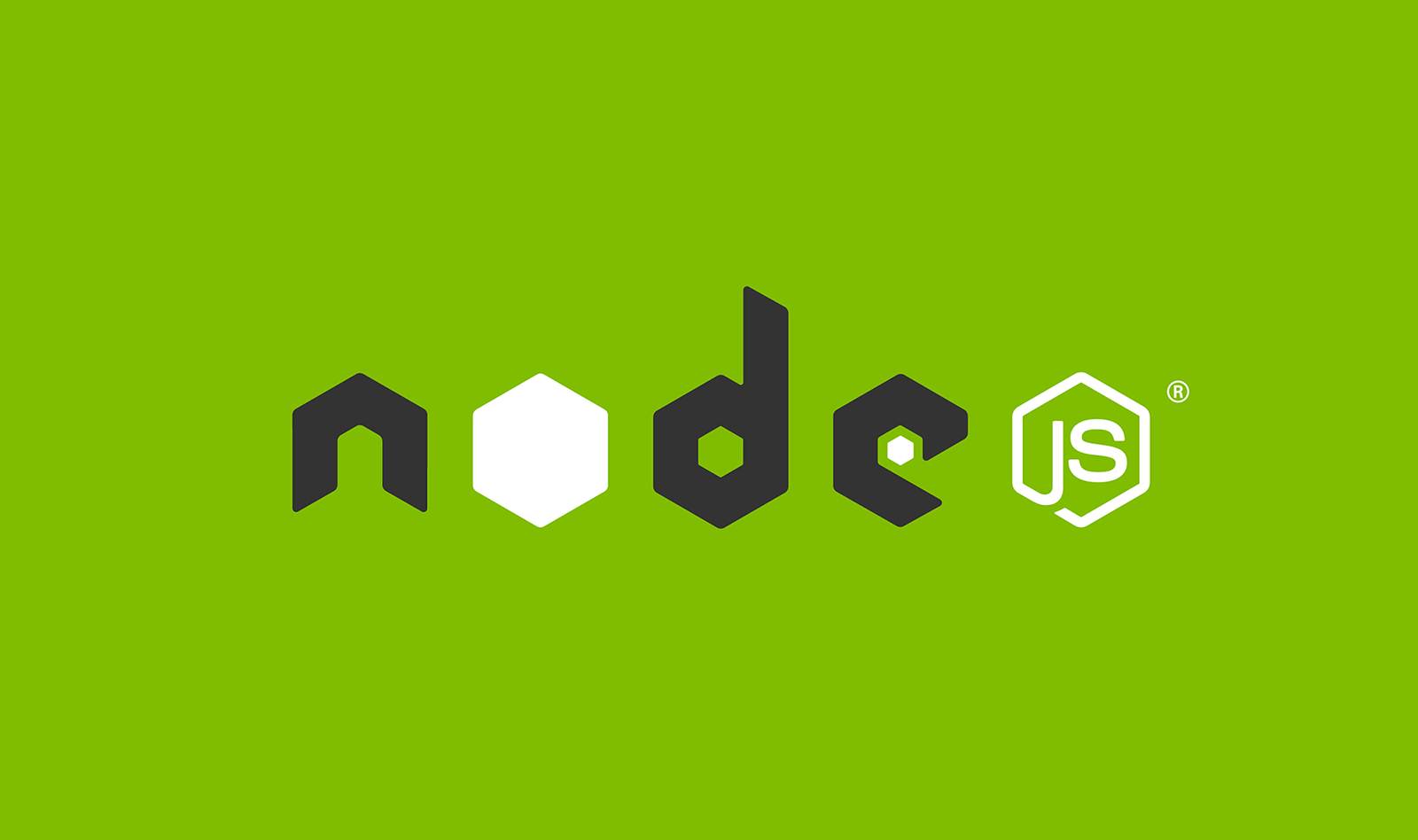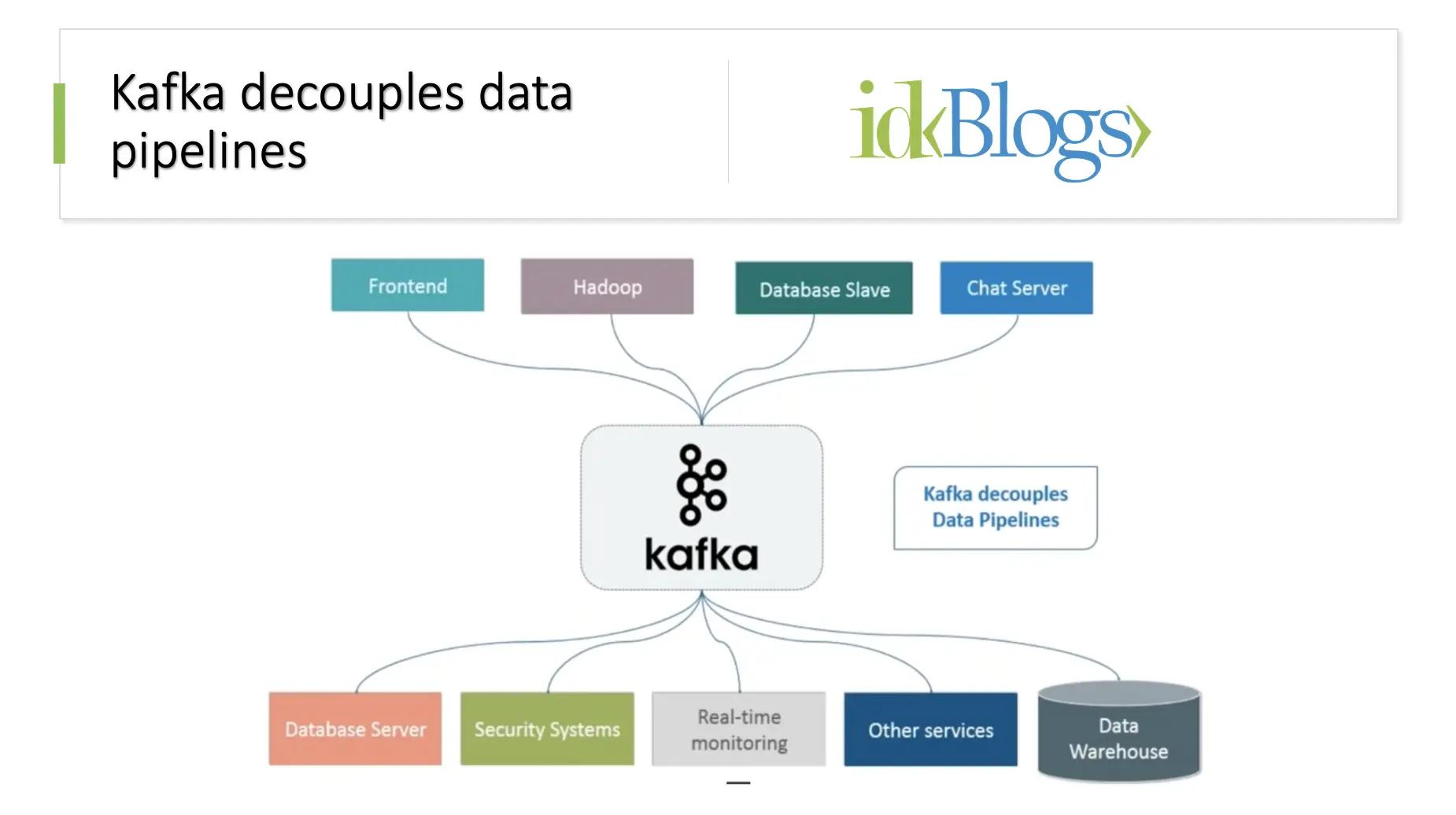# What are the CPU intensive tasks?

## CPU-intensive tasks are operations that heavily rely on the computational power of the CPU.

🙋‍♂️ Shubham Verma    🗓 May 21, 2023## What are the CPU intensive tasks?

In the world of computing, certain tasks require significant processing power from the CPU (Central Processing Unit). These tasks, known as CPU-intensive tasks, demand extensive computational resources and can put a strain on the processor. Understanding CPU-intensive tasks is essential for optimizing performance and resource allocation in software development and system administration. In this article, we will explore what CPU-intensive tasks are, examples of such tasks, and considerations for efficiently handling them.

CPU-intensive tasks are operations that heavily rely on the processing power of the CPU rather than other system resources. These tasks typically involve complex calculations, mathematical operations, simulations, or data processing that require significant computational resources. Unlike I/O-bound tasks that wait for input/output operations, CPU-intensive tasks focus on executing instructions and manipulating data within the CPU.

1. Cryptocurrency Mining: The process of mining popular cryptocurrencies, such as Bitcoin or Ethereum, involves solving complex mathematical puzzles that demand extensive computational power.

2. Video Rendering and Encoding: Converting raw video footage into compressed formats, rendering computer-generated imagery (CGI), or applying special effects to videos requires intensive CPU calculations.

3. Scientific Simulations: Tasks in scientific research, such as simulating weather patterns, molecular dynamics, or nuclear simulations, often involve complex mathematical calculations that are computationally demanding.

4. Data Analytics and Machine Learning: Performing complex data analytics, statistical analysis, or training and running machine learning models on large datasets can be highly CPU-intensive.

5. Genetic Sequencing: Analyzing DNA sequences, conducting genetic research, or running bioinformatics algorithms involve substantial computational processing to identify patterns, mutations, or genetic markers.

### Efficient Handling of CPU-Intensive Tasks

To effectively handle CPU-intensive tasks, consider the following strategies:

1. Parallelization: Distribute the workload across multiple CPU cores or utilize technologies like multithreading or multiprocessing to leverage the full potential of the available processing power.

2. Optimized Algorithms: Implement efficient algorithms and data structures that minimize redundant calculations and maximize computational efficiency.

3. Hardware Acceleration: Utilize specialized hardware, such as graphics processing units (GPUs) or field-programmable gate arrays (FPGAs), to offload and accelerate CPU-intensive computations.

4. Caching and Memoization: Cache intermediate results or implement memoization techniques to avoid redundant calculations and optimize the use of CPU resources.

5. Load Balancing: Distribute CPU-intensive tasks across multiple machines or instances to achieve better load distribution and prevent resource bottlenecks.

### Code Examples in Node.js:

Here are a few code snippets in Node.js to illustrate CPU-intensive tasks:
``````                            // Example 1: Fibonacci Sequence Calculation
function fibonacci(n) {
if (n <= 1) return n;
return fibonacci(n - 1) + fibonacci(n - 2);
}
console.log(fibonacci(10));

// Example 2: Prime Number Calculation
function isPrime(num) {
for (let i = 2, sqrt = Math.sqrt(num); i <= sqrt; i++) {
if (num % i === 0) return false;
}
return num > 1;
}
console.log(isPrime(17));

// Example 3: Matrix Multiplication
function matrixMultiply(matrixA, matrixB) {
// Implementation logic goes here
return resultMatrix;
}
const matrixA = [[1, 2], [3, 4]];
const matrixB = [[5, 6], [7, 8]];
console.log(matrixMultiply(matrixA, matrixB));
``````

#### Conclusion:

CPU-intensive tasks are an integral part of various computational domains, ranging from scientific research to video rendering and data analytics. Understanding these tasks and implementing efficient strategies for handling them is crucial for optimizing performance and resource utilization. By leveraging parallelization, optimized algorithms, hardware acceleration, caching, and load balancing techniques, developers and system administrators can effectively tackle CPU-intensive tasks and achieve optimal performance in their applications and systems.

### Related Keywords:

#### Support our IDKBlogs team

Creating quality content takes time and resources, and we are committed to providing value to our readers. If you find my articles helpful or informative, please consider supporting us financially.

Any amount (10, 20, 50, 100, ....), no matter how small, will help us continue to produce high-quality content.

Thank you

I appreciate you taking the time to read this article. The more that you read, the more things you will know. The more that you learn, the more places you'll go. If you’re interested in Node.js or JavaScript this link will help you a lot.

To read more about the technologies, Please subscribe us, You'll get the monthly newsletter having all the published article of the last month.

### OTHER POSTS